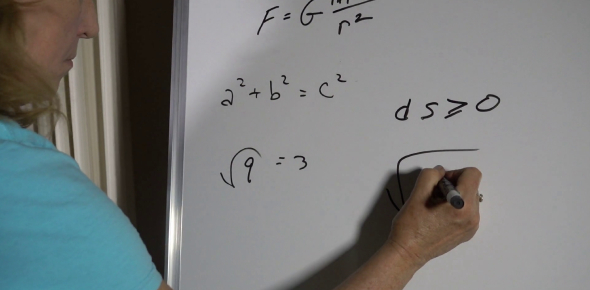# Writing Linear Equations

15 QuestionsSettingsRelated Topics
• 1.
Which is the slope - intercept form of a line?
• A.

M = yx + b

• B.

M =(y2-y1) / (x2-x1)

• C.

Y = mx + b

• D.

Y = bx + m

• 2.
What is the slope of the following line? 3x + 7y = 4
• 3.
Determine the slope from the following two points(3,6) and (5,-2)
• 4.
Determine the slope from the following two points.(0,2) and (-4,-2)
• 5.
Rewrite in slope-intercept form.4x - 2y = 6
• 6.
Write the equation in slope-intercept form of the line passing through (-2,4) and has a slope of 3.
• 7.
What is the x-intercept of the equation -2x + 9y = 12
• A.

12/9

• B.

6

• C.

-6

• D.

None of the above

• 8.
Find the equation of a line passing through (0, 3) and with a slope of 1/2
• A.

Y = 1/2x + 3

• B.

Y = 2x + 3

• C.

Y = -2x + 3

• D.

Y = 3x + 1/2

• E.

Not enough information

• 9.
Write the equation of a line passing through points (4, 5) and (2, 8).
• A.

Y = 3/2x - 1

• B.

Y = 2/3x + 1

• C.

Y = -3/2x + 11

• D.

Y = -3/2x - 1

• E.

Y = 3/2x + 5

• 10.
Find the equation of a line passing through (2, 6) and (3, 9).
• A.

Y = -3x + 12

• B.

Y = 3x

• C.

Y = -3x

• D.

Y = 1/3x +5 1/3

• E.

None of the above

• 11.
Find the equation of a line passing through (4, 7) and (-2, 10).
• A.

Y = 1/2x + 5

• B.

Y = -1/2x - 6

• C.

Y = 2x - 1

• D.

Y = -1/2x + 9

• E.

Y = -2x + 6

• 12.
What is the equation of the line passing through (2, 3) and (2, 8)?
• A.

X = 2

• B.

Y = 2

• C.

Y = 5x

• D.

None of the above

• 13.
What is the equation of the line passing through (9, 1) and (-5, 1)?
• A.

X = 1

• B.

Y = 1

• C.

Y = 14

• D.

X = 14

• E.

None of the above

• 14.
What is the solution of -20 = g - 4?
• A.

-24

• B.

-16

• C.

5

• D.

80

• 15.
What is the solution of the equation?  -4n - 20 = -32
• A.

3

• B.

13

• C.

-13

• D.

-12